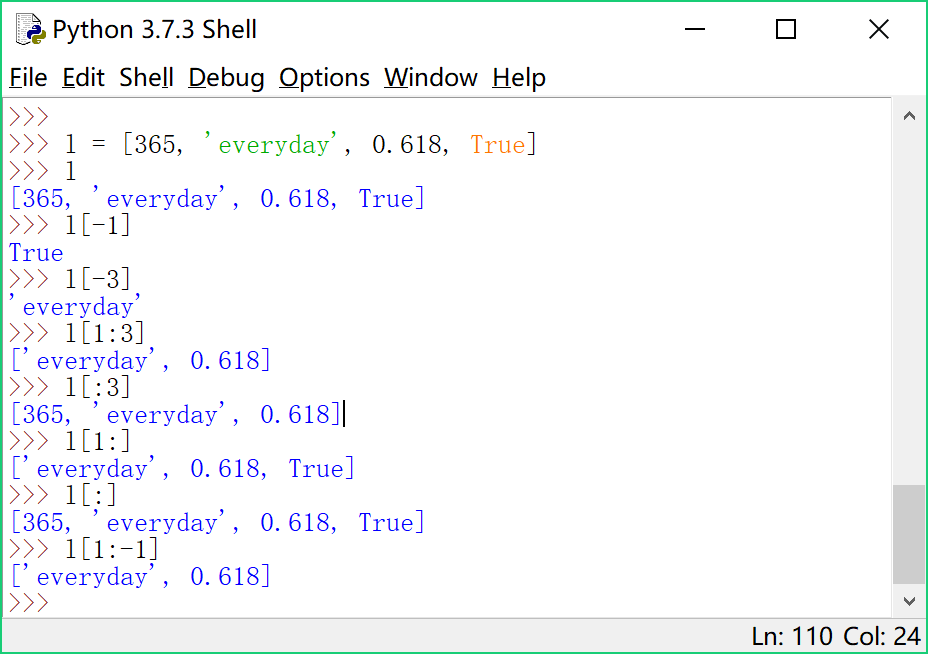## 【Python 第27课】list切片

list有两类常用操作：索引(index)切片(slice)

``l = [365, 'everyday', 0.618, True]``

l[-1]表示l中的最后一个元素。

l[-3]表示倒数第3个元素。

``l[1:3]``

``````l[:3]
l[1:]
l[:]``````

``l[1:-1]``#==== 点球小游戏 ====#

``````from random import choice

score_you = 0
score_com = 0
direction = ['left', 'center', 'right']

for i in range(5):
print ('==== Round %d - You Kick! ====' % (i+1))
print ('Choose one side to shoot:')
print ('left, center, right')
you = input()
print ('You kicked ' + you)
com = choice(direction)
print ('Computer saved ' + com)
if you != com:
print ('Goal!')
score_you += 1
else:
print ('Oops...')
print ('Score: %d(you) - %d(com)\n' % (score_you, score_com))

print ('==== Round %d - You Save! ====' % (i+1))
print ('Choose one side to save:')
print ('left, center, right')
you = input()
print ('You saved ' + you)
com = choice(direction)
print ('Computer kicked ' + com)
if you == com:
print ('Saved!')
else:
print ('Oops...')
score_com += 1
print ('Score: %d(you) - %d(com)\n' % (score_you, score_com))``````